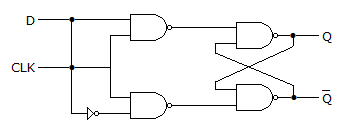# Electronics and Communication Engineering - Digital Electronics

1.

Assertion (A): A demultiplexer can be used as a decoder.

Reason (R): A demultiplexer can be built by using AND gates only.

 A. Both A and R are correct and R is correct explanation of A B. Both A and R are correct but R is not correct explanation of A C. A is true, R is false D. A is false, R is true

Explanation:

Demultiplexer requires NOT gates also in addition to AND gates.

2.

Assertion (A): The output of a NOR gate is equal to the complement of OR of input variables.

Reason (R): A XOR gate is a universal gate.

 A. Both A and R are correct and R is correct explanation of A B. Both A and R are correct but R is not correct explanation of A C. A is true, R is false D. A is false, R is true

Explanation:

XOR gate is not a universal gate.

3.

The number of bits in ASCII is

 A. 12 B. 10 C. 9 D. 7

Explanation:

ASCII is a 7 bit code.

4.

4 bit 2's complement representation of a decimal number is 1000. The number is

 A. + 8 B. 0 C. - 7 D. - 8

Explanation:

(a) and (d) both are option, But there is meaning to represent a positive number in 2's complement form, we take complement representation for negative number only. Therefore most appropriate number is "-8".

5.

In a D latch

 A. data bit D is fed to S input and D to R input B. data bit D is fed to R input and D to S input C. data bit D is fed to both R and S inputs D. data bit D is not fed to any input